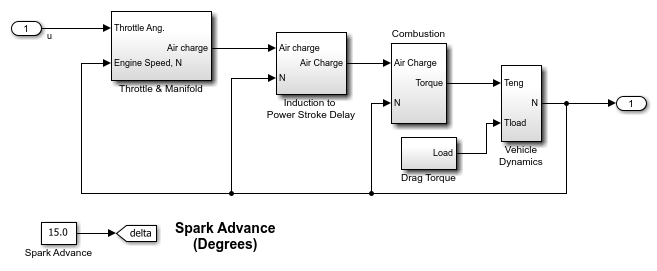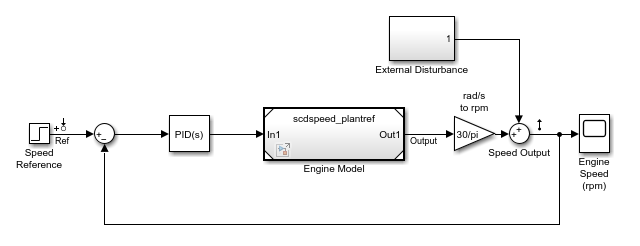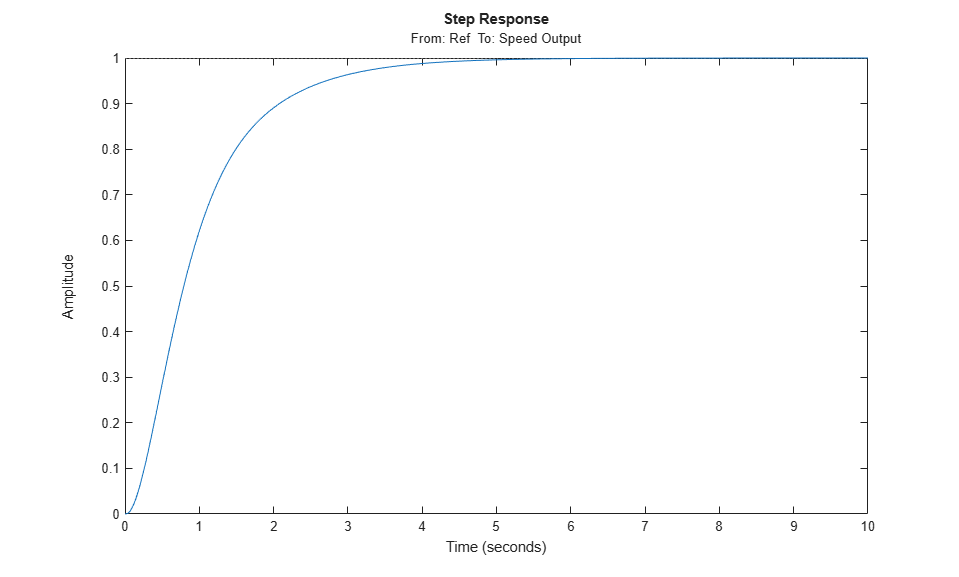# Linearize Models with Model References

This example shows how to linearize models that include references to other models using a Model block.

The `scdspeed_ctrlloop` model is a modified version of the `scdspeedctrl` model.

```topmdl = 'scdspeed_ctrlloop'; open_system(topmdl) ```For this example, the engine speed system is implemented in the `scdspeed_plantref` model. This model is referenced in the `scdspeed_ctrlloop` using a Model block.

View the referenced engine speed model.

```open_system('scdspeed_plantref') ```By default, the Engine Model block is set to accelerator simulation mode, as indicated by the black triangles at the corners of the Model block. Linearizing the model with this block in accelerator mode numerically perturbs the entire Engine Model block. The accuracy of this linearization is sensitive to the blocks within the Engine model. In particular, the variable transport delay block is problematic.

To achieve an accurate linearization, set the Model block to normal simulation mode, which enables the block-by-block linearization of the referenced model.

```set_param('scdspeed_ctrlloop/Engine Model','SimulationMode','Normal') ```

The corners of the Engine Model block are now white triangles, indicating that its simulation mode is set to normal.Linearize the model between the speed reference signal and the speed output, and plot the resulting step response.

```io(1) = linio('scdspeed_ctrlloop/Speed Reference',1,'input'); io(2) = linio('scdspeed_ctrlloop/Speed Output',1,'output'); sys_normal = linearize(topmdl,io); step(sys_normal) ```Another benefit of switching the model reference to normal mode simulation is that you can take advantage of exact delay representations. For more information on linearizing models with delays, see Linearize Models with Delays.

```bdclose('scdspeed_ctrlloop') ```